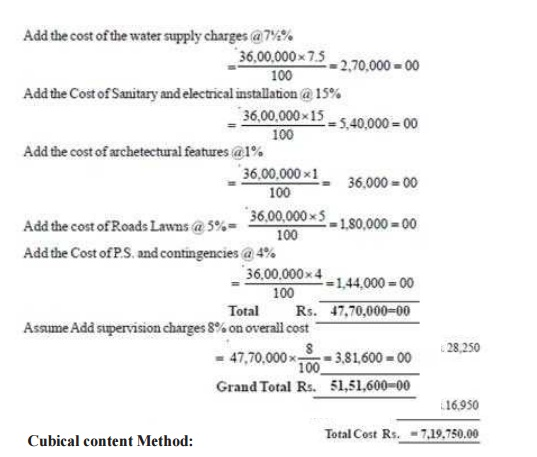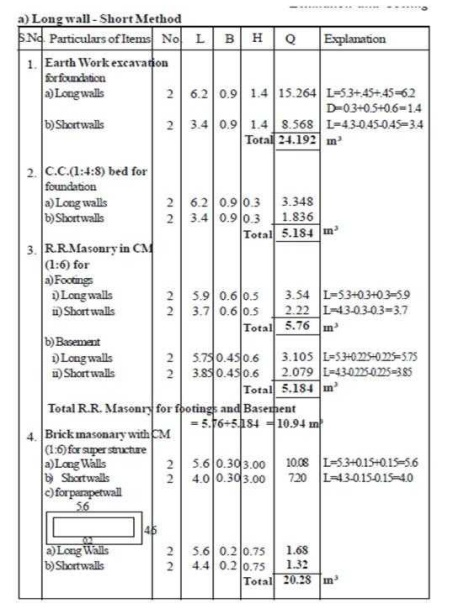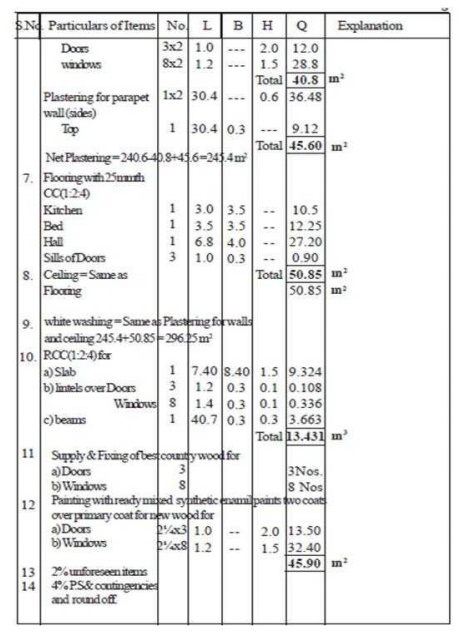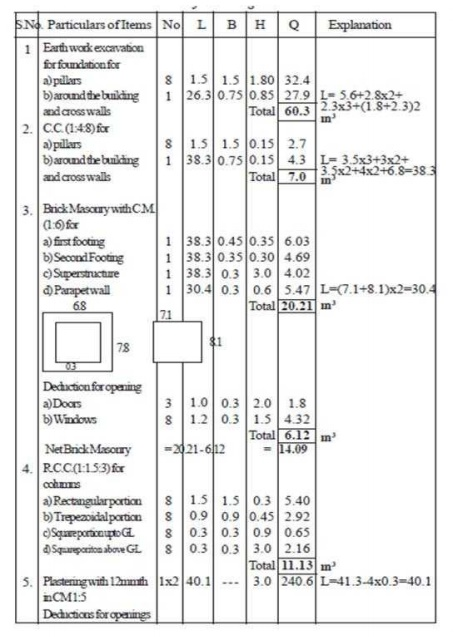Home | | Estimation and Quantity Surveying | Design Problems: Civil - Estimation and Quantity Surveying - Estimate of Buildings

# Design Problems: Civil - Estimation and Quantity Surveying - Estimate of Buildings

Civil - Estimation and Quantity Surveying - Estimate of Buildings

Example 1 .1: Prepare an approximate estimate of building project with totalplinth area of all building is 800 sqm. and from following data.

i) Plinth area rate Rs. 4500 per sqm

ii) Cost of water supply @7� %of cost of building.

iii)  Cost of Sanitary andElectrical installations each @ 7�%  of cost ofbuilding.

iv)Cost of architectural features @1% of building cost.

v)Cost of roads and lawns @5% of building cost.

vi)    Cost  of  P.S.  and  contingencies  @4%  of  building

cost. Determine the total cost of building project.

Solution:

Data given:

Plinth area = 800m2

Plinth area rate = Rs. 4500 per Sq.m

Cost of building = 800 x 4500 = Rs. 36,00,000=00Example 1 . 2 :Prepare the rough estimate for a proposed commercial complexfor a municipal corporation for the following data.

Plinth Area = 500m2/floor Ht of each storey = 3.5m No. of storey's = G+2 Cubical content rate = Rs. 1000/m3

Provided for a following as a percentage of structured cost

a) water supply & Sanitary arrangement -8%

b)Electrification -6%

c) Fluctuation of rates - 5%

d)Contractors profit - 10%

e)Petty supervision & contingencies - 3%

Sol :

Cubical content = No. of storey's (Plinth Area x height of each storey) = 3(500x3.5) = 5250m3

Structural cost = Cubical content x cubical content rate= 5250 x 1000 = 52.5 LakhsUnit Base Method

Example 1.3: Prepare an approximate estimate or rough cost estimate of ahospital building for 50 beds. The cost of construction altogether for each bed isRs. 60,000/ -. Determine the total cost of hospital building.

Solution:

No. of beds = 50

Cost of construction = Rs. 60,000/-

Total Cost of Hospital building = 50x 60,000= Rs. 30,00,000/-

Example 1.4: To prepare the rough cost estimate of a hostel building whichaccommodate 150 students. The cost of construction including all provisions isRs. 15,000/- per student. Determine total cost of building.

Solution :

No.of students= 150

Cost of construction including all L.S. provisions = Rs. 15,000/- Total Cost of hostel building =150 x 15000 = Rs. 22,50,000/- (Rupees twenty two lakhs, fifty thousand only)

Example 1 . 5 : From the given figure below calculate the detailed and abstract estimate for the single roomed building (Load bearing type structure) by

a)   long wall & short wall method

b)  Centre Line MethodExample 1.6 From the given figure below calculate the details and abstractestimate for the single Storied residential buildingwith no of rooms (Load bearing type structure) by Centre lineExample 1.7 From the given figure below calculate the details and abstractestimate for the single storied residential building withno. of rooms (Framed Structured type) by Centre Line MethodStudy Material, Lecturing Notes, Assignment, Reference, Wiki description explanation, brief detail
Civil : Estimation and Quantity Surveying : Estimate of Buildings : Design Problems: Civil - Estimation and Quantity Surveying - Estimate of Buildings |